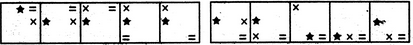# Non Verbal Reasoning - Series - Discussion

Discussion Forum : Series - Section 1 (Q.No. 20)
Directions to Solve

Each of the following questions consists of five figures marked A, B, C, D and E called the Problem Figures followed by five other figures marked 1, 2, 3, 4 and 5 called the Answer Figures. Select a figure from amongst the Answer Figures which will continue the same series as established by the five Problem Figures.

20.

Select a figure from amongst the Answer Figures which will continue the same series as established by the five Problem Figures.(A)     (B)     (C)     (D)     (E)       (1)     (2)     (3)     (4)     (5)
1
2
3
4
5
Explanation:
In the first step, the ACW end element moves two spaces (each space is equal to half-a-side of the square boundary) in an ACW direction. In the second step, the CW-end element moves three spaces ACW. In the third step, the remaining element moves four spaces ACW. The three steps are repeated to continue the series.
Discussion:
13 comments Page 1 of 2.

Eve Che said:   10 months ago
I think 4 is the answer.

Star is 2 steps ACW.
x is 3 steps ACW.
= is 4 steps CW.

Jatin Sharma said:   12 months ago
How do you find the ACW end and CW end elements? Please explain.

Anjali said:   1 year ago
I'm not getting it. Please anyone help me to get this.

Ankit said:   5 years ago
Thank you @Lucy.

Aravindh said:   6 years ago
Thank you @Jim.

Lucy said:   7 years ago
1. Move two steps.
2. Move three steps.
3. Move four steps.

Cedric Charles said:   7 years ago
@Jim.

Your explanation is crystal clear, Thank you.

Jim said:   10 years ago
Elements move in ACW direction, keep this in mind.

1). The ACW end element moves two EMPTY spaces (space being a half-side as in the explanation).

2). The CW end element moves two EMPTY spaces.

3). The REMAINING element moves two EMPTY spaces.

The above three steps are repeated.

After applying one cycle of the above steps we are in figure (D).

For the next cycle, the ACW end element is "=", which has been moved two EMPTY spaces, resulting in figure (E).

From figure (E) , the CW end is "x", which has to be moved two EMPTY spaces. This results in figure 5.

1st Step * moves 1 step ACW.

2nd Step X moves 1.5 step ACW(in ACW X is next to *).

3rd Step = moves 2 Step ACW.

In this Series in Fig 5,
= moves 1 Step ACW.

Similarly X Moves 1.5 Step. (in ACW X is next to =).